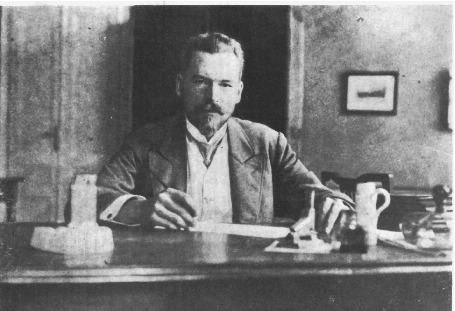#33rd M. Smoluchowski Symposium on Statistical Physics

Dec 3 – 4, 2020
Europe/Warsaw timezone

## Energetics of critical oscillators in active bacterial baths

Dec 3, 2020, 6:07 PM
1m
poster

### Speaker

Ashwin Gopal (University of Luxembourg)

### Description

We investigate the nonequilibrium energetics near a critical point of a non-linear driven oscillator immersed in an active bacterial bath. At the critical point, we reveal a scaling exponent of the average power $\langle\dot{W}\rangle\sim (D_{\rm a}/\tau)^{1/4}$ where $D_{\rm a}$ is the effective diffusivity and $\tau$ the correlation time of the bacterial bath described by Gaussian colored noise. Other features that we investigate are the average stationary power and the variance of the work both below and above the saddle-node bifurcation. Above the bifurcation, the average power attains an optimal, minimum value for finite $\tau$ that is below its zero-temperature limit. Furthermore, we reveal a finite-time uncertainty relation for active matter, which leads to values of the Fano factor of the work that can be below $2k_{\rm B}T_{\rm eff}$, with $T_{\rm eff}$ the effective temperature of the oscillator in the bacterial bath. We analyze different Markovian approximations to describe the nonequilibrium stationary state of the system. Finally, we illustrate our results in the experimental context by considering the example of driven colloidal particles in periodic optical potentials within a E. Coli bacterial bath.

### Primary authors

Ashwin Gopal (University of Luxembourg) Edgar Roldan (Max Planck Institute for the Physics of Complex Systems) Stefano Ruffo (SISSA)

### Presentation materials

There are no materials yet.